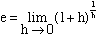Subject: Natural Logarithm Name: Shimin Who is asking: Student Level: Secondary Question: Hi! I would like to ask about the exact definition of e, its history (like how John Napier came about discovering it) and its applications in problems and real life situations. Thank you! Hi Although one commonly reads that Napier's logarithms were the "natural" logarithms (to base e), the fact is that his invention was only remotely related to the natural logarithm. Napier's ideas would be familiar to a student of calculus, but because his work was done (published in 1614) some 75 years before the invention of calculus, it was much more complicated than it might have been. Napier realized shortly before his death in 1617 that a base 10 system would be more practical for computations, so it fell to one of his followers, Henry Briggs, to prepare a table of "common" logarithms.    The way to see that "e" arises from nature, you have to understand something about exponential growth. An exponential growth law says that the rate of increase is proportional to the amount present. (Think of a human population -- the more people there are, the more children there will be.) The functions that satisfy such a growth law (normalized so that their value is 1 when x = 0) must have the form c^x for some number c. When e^x is used, the law states that the growth rate EQUALS the amount present (in symbols, y' = y). From this fact one can deduce that e is(To get a feeling for what this means, just replace h by a small number such as .001.) An easier definition is e = 1/1 + 1/(1*2) + 1/(1*2*3) + ... + 1/(n!) + ... . You stop taking the sum when you get the accuracy you want. A place to find more information is The MacTutor History of Mathematics archive and in particular their information on Napier. Cheers Chris

Go to Math Central

To return to the previous page use your browser's back button.